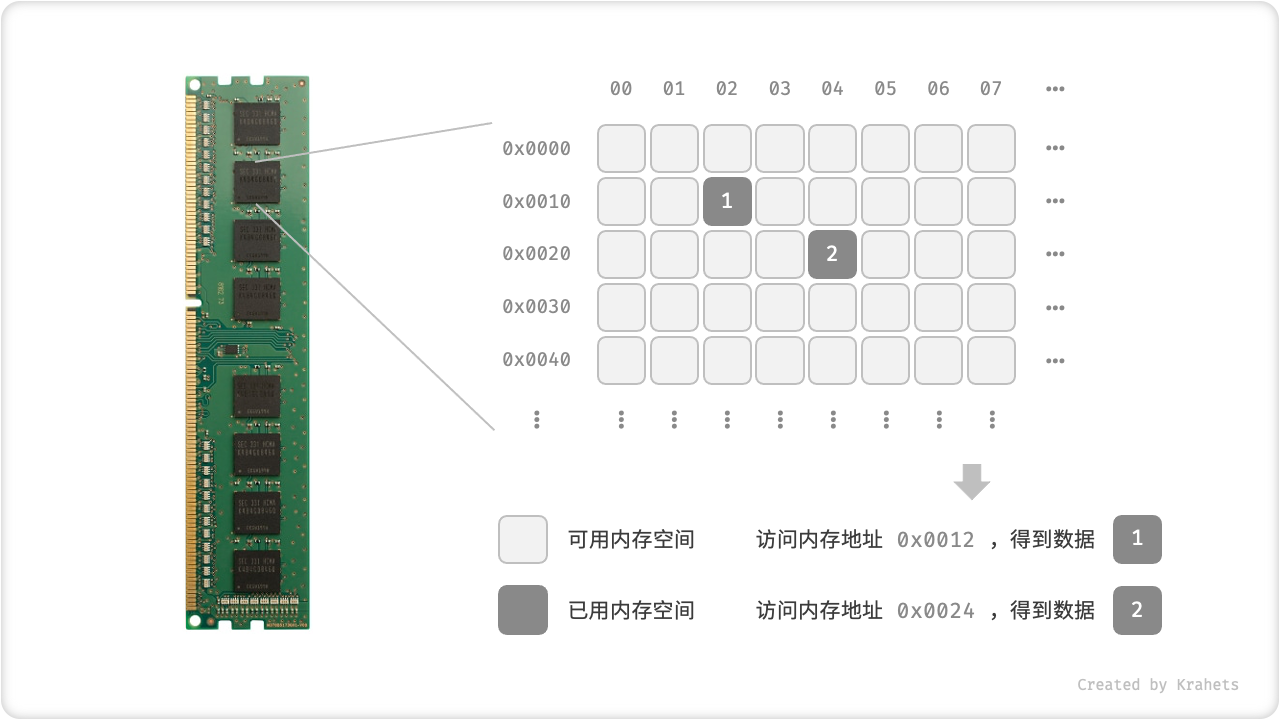# 数据与内存¶

## 基本数据类型¶

「基本数据类型」是 CPU 可以直接进行运算的类型，在算法中直接被使用。

• 「整数」根据不同的长度分为 byte, short, int, long ，根据算法需求选用，即在满足取值范围的情况下尽量减小内存空间占用。
• 「浮点数」代表小数，根据长度分为 float, double ，同样根据算法的实际需求选用。
• 「字符」在计算机中是以字符集的形式保存的，char 的值实际上是数字，代表字符集中的编号，计算机通过字符集查表来完成编号到字符的转换。
• 「布尔」代表逻辑中的 ”是“ 与 ”否“ ，其占用空间需要具体根据编程语言确定，通常为 1 byte 或 1 bit 。

1 字节 (byte) = 8 比特 (bit) ， 1 比特即最基本的 1 个二进制位

Table. Java 的基本数据类型

short 2 bytes $$-2^{15}$$ ~ $$2^{15} - 1$$ $$0$$
int 4 bytes $$-2^{31}$$ ~ $$2^{31} - 1$$ $$0$$
long 8 bytes $$-2^{63}$$ ~ $$2^{63} - 1$$ $$0$$

double 8 bytes $$-1.7 \times 10^{308}$$ ~ $$1.7 \times 10^{308}$$ $$0.0$$

Tip

「基本数据类型」与「数据结构」之间的联系与区别

/* 使用多种「基本数据类型」来初始化「数组」 */
int[] numbers = new int;
float[] decimals = new float;
char[] characters = new char;
boolean[] booleans = new boolean;
















## 计算机内存¶Fig. 内存条、内存空间、内存地址